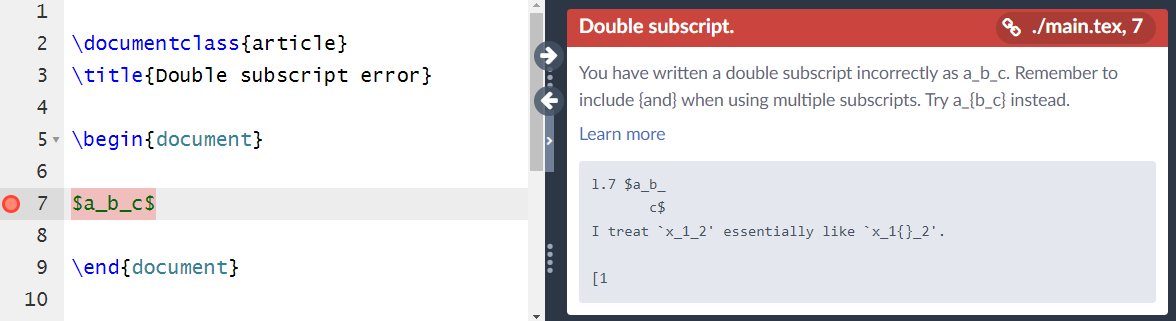Aller au contenu

## Introduction

By convention, LaTeX uses the underscore character (_) to typeset a subscript, such as $a_b$ which produces $a_b$. If you write $a_bcde$ only the first character, b, is subscript, $a_bcde$, because LaTeX does not assume you want to subscript the entire set of characters bcde. To typeset multiple items (tokens) as subscripts, you need to tell LaTeX by enclosing them in a group {...}, like this: $a_{bcde}$, which produces $a_{bcde}$.

## Double subscript error

The double subscript error arises when LaTeX is asked to add a subscript to a piece of mathematics which already has a subscript attached to it. This error is usually resolved through the use of braces {...} which, within math mode, generate a so-called subformula—a term used to describe a fragment of the mathematical expression you are trying to typeset.

For example, writing $a_b_c$ generates the following double subscript error:This particular error can be fixed in several ways through the use of braces {...}—the results vary according to where the braces are positioned:

• $a_{b_c}$ typesets $\displaystyle a_{b_c}$
• ${a_b}_c$ typesets ${a_b}_c$
• $a_{bc}$ typesets $a_{bc}$

### Double subscript error: a special case

A question on tex.stackexchange highlights a surprising example of the double subscript error, one whose cause and corresponding fix may be worth knowing about. We reproduce the example here.

The following example fails to compile, even though braces are used:

\documentclass{article}
\begin{document}
$${\vec a_b}_c$$
\end{document}


One way to fix the problem is to add the accents package to your document preamble (as suggested here):

\documentclass{article}
\usepackage{accents}
\begin{document}
$${\vec a_b}_c$$
\end{document}


## More on subscripts

Further levels of subscripts can be typeset by writing LaTeX such as $a_{b_{c_{d_e}}}$ which typesets $a_{b_{c_{d_e}}}$. All subscripts at or below the second level (sub-subscript) are typeset using the same font size (in points), whereas first-level subscripts are typeset using a slightly larger font (point size). To learn more about subscripts, visit Overleaf's help page dedicated to that topic.